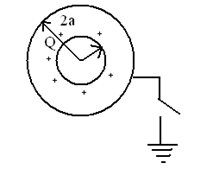Potential energy
Question

# Two conducing concentric hollow spheres of radii a and 2a respectively are shown in the diagram.  The inner shell has net charge Q on its surface.  The outer shell is neutral.  If the outer shell is connected to the earth the heat generated through the connecting wire isModerate
Solution

## Outside energy $=\frac{1}{4\mathrm{\pi }{\in }_{0}}\left(\frac{{\mathrm{Q}}^{2}}{2×2\mathrm{a}}\right)$  will become zero as the shell is earthed.  This energy appears as heat

Get Instant Solutions